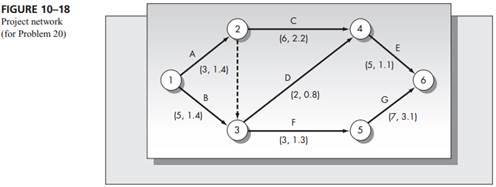Create an Account

Home / Questions / Consider the project network pictured in Figure 10–18 Assume that the times attached to e...

Consider the project network pictured in Figure 10–18 Assume that the times attached to each node are the means and the variances of the project completion times respectively

Consider the project network pictured in Figure 10–18. Assume that the times attached to each node are the means and the variances of the project completion times, respectively.

a. Identify all paths from nodes 1 to 6.

b. Which path is critical based on the expected completion times?

c. Determine the probability that the project is completed within 20 weeks assuming that the path identified in part (b) is critical.

d. Using independence of paths A–C–E and B–F–G only, recompute the answer to part (c).

e. Recompute the answer to part (c) assuming independence of the paths identified in part (a).

f. Which answer, (c), (d), or (e), is probably the most accurate?Jul 27 2020 View more View LessSubscribe To Get Solution